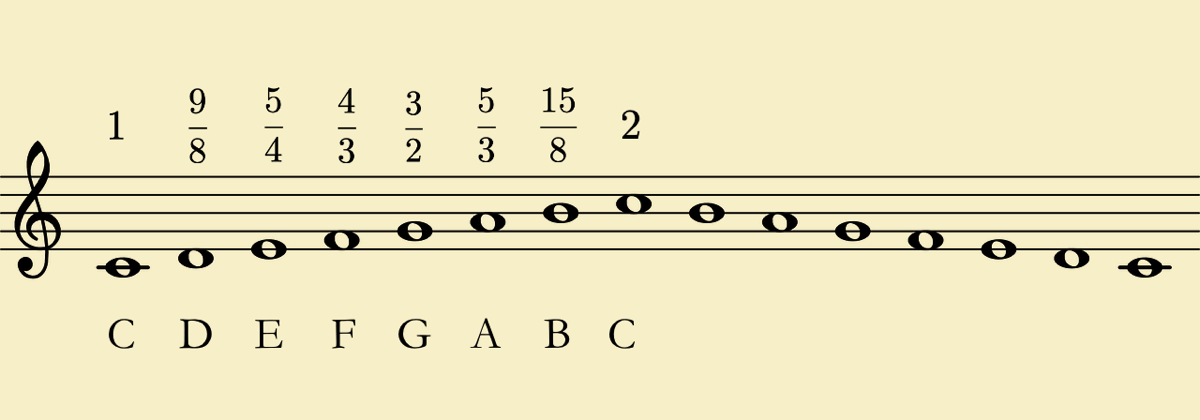# The Blog of Mathedu Academy

### A content for the curious of the mathematics in the real world… and beyond# Music and Math: how is the Major C scale built?

The Greeks discovered the link between the musical intervals and the length of a string. The simpler example is that half the string sounds at the octave of the whole string. We know now that this corresponds to twice the frequency of the vibration.

Thus, 2 sounds differing by a 2 ratio on their vibrations have an interval of an octave. This is why the octave sounds so well to our ears, and was chosen as the periodicity of the scale.

The next such "harmonic" is when we divide the string in 3 equal parts, or else multiply the frequency by 3. Then we obtain the fifth of the octave and, if we take the double of that length (2/3 of the total length, or 3/2 of the frequency), we obtain a "perfect" fifth.

The fifth sounds also very good to our ears, because its frequencies are in a a simple ratio: 3/2 is done with small numbers.

But let us now focus on the major C scale. We know now that the fifth of C, that is G, is at 3/2 the frequency of C.

Then the major third of C, that is E, has a frequency with ratio 5/4 with the frequency of C. This is because the 5th "harmonic" of a sound (multiply the frequency by 5, or divide the string by 5) is 2 octaves above the major third.

With these three notes, C, E and G, we obtain the "Tonic Chord" of C, called "perfect" is French, because it sounds so well to ours ears.

We may continue like that and construct al the major C scale, with only simple fractional ratios between the frequencies of the notes of the scale and the frequency $$f_0$$ of C0:
• C0 is $$1 \times f_0$$, the fundamental
• D is $$\frac{9}{8} \times f_0$$, the major second
• E is $$\frac{5}{4} \times f\_0$$, the major third
• F is $$\frac{4}{3} \times f\_0$$, the fourth
• G is $$\frac{3}{2} \times f_0$$, the fifth
• A is $$\frac{5}{3} \times f\_0$$, the major sixth
• B is $$\frac{15}{8} \times f\_0$$, the major seventh
• and C1 is $$2 \times f\_0$$, the octave

Note that C0 and C1 are any two Cs, with C1 at the octave of C0.

And with that, we may calculate the frequencies ratio for a minor fifth, between A and C, 2 divided by $$\frac{5}{3}$$, that is $$\frac{6}{5}$$, or else between E and G, $$\frac{3}{2}$$ divided by $$\frac{5}{4}$$, that is $$\frac{12}{10} = \frac{6}{5}$$ as well, because it is another minor fifth.

Thus, the minor third sounds also good to our ears, because of that simple ratio.

And this is the first step of musical construction in concordance with mathematics.

It is only the beginning, because with constructed only the Major C scale. And if we want to construct other scales, we should discover the sharp and flat notes… and that we can not continue with simple ratios for all the tones!

But this is another story…
Back